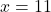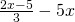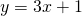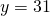# Solve Linear Equations

An equation is a numerical statement that has an ‘=’ sign.

Example:One of the numbers has been written as a letter. We solve an equation when we calculate the number that has been written as a letter.

Since we know thatwe can say that. The numberis the solution to the equation above.

Equations come in many forms.

Linear equations are equations that we solve by adding, subtracting, multiplying or dividing only.

Here are some examples (to be completed):

 One Step add subtract multiply divide twostep example multiple steps linear linear with brackets linear linear with many brackets linear linear with one fraction linear linear with more than one fraction linear linear special case fraction equal fraction linear linear

# Vocabulary

 Examples term;;;;;expression;equation;relationA term is a number or an unknown, or a product (multiplication) of numbers/unknowns. A term might also be the product of expressions, such as.

An expression is the addition (or subtraction) of one or more terms.

An equation puts a condition on an expression. For example. Although we callan unknown, in an equation we can usually figure out what it is. That’s the process of solving an equation.

A relation is when we introduce a second unknown. For example,. Now, should we give thea value, then thewill have a value too and vice versa. Suppose we say that. That means that. If you draw a graph of a linear expression, you get a straight line, which is where the name ‘linear’ comes from. In relations, unknowns are generally referred to as variables.

# Identifying a Solution to a Linear Equation by Substitution

As an introduction to algebra, an equation might look like this:The idea is that you’re supposed to find the number that goes in the box to make the calculation correct.

The big idea of algebra is to use a letter to represent the uknown number until you figure it out (in an equation) or give it a value (in a relation). That is:Which we shorten to:In the following applet, you are given three possible solutions. Which number makes the calculation correct? Figure it out by replacing thewith one of the numbers given until you find one that fits – or your own strategy.

# Solving linear equal a constant

Mathantics Scroll down to ‘Algebra Basics Part 1’ for a set of four videos on solving equations.

# Solving Linear equal Linear Equation

It can be helpful to follow some predetermined steps to solve these.

• Expand any brackets, simplify each side if necessary;
• Add or subtract theterm from one side so that there is anterm on one side only;
• Continue as before, in skill 4.

There could be a better approach than these three steps – it really depends on the equation you’re solving. This is why it is good to keep thinking about the objective (figuring out whatworks) and not get too hung up on one method.

# When there are fractions

Albegraic problems with fractions show up in the solutions to problems in topics 2, 4, 5, 6 and 7. Invest some time here, and keep coming back until this is something you’ve mastered!

Example Solve with Fractions

# More!

Try these algebra problems from an 1857 textbook!